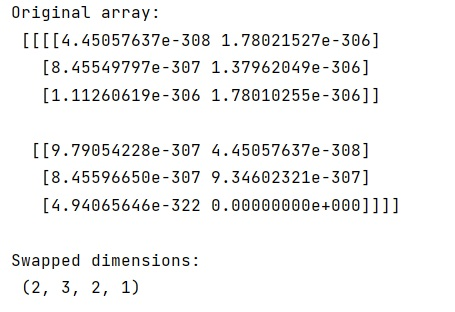# Swapping the dimensions of a NumPy array

Learn how to swap the dimensions of a NumPy array in Python?
Submitted by Pranit Sharma, on February 11, 2023

NumPy is an abbreviated form of Numerical Python. It is used for different types of scientific operations in python. Numpy is a vast library in python which is used for almost every kind of scientific or mathematical operation. It is itself an array which is a collection of various methods and functions for processing the arrays.

## NumPy Array - Swapping the Dimensions

Suppose that we are given a NumPy array that contains some integer values and we need to swap the dimensions of this array. We can use the transpose of the empty matrix and it will change the shape of the array so that the dimensions can be swapped. We will use numpy.transpose() optional permutation argument.

Let us understand with the help of an example,

## Python code to swap the dimensions of a NumPy array

```# Import numpy
import numpy as np

# Creating an empty matrix of some dimension
a = np.empty((1, 2, 3, 2))

# Display original array
print("Original array:\n",a,"\n")

# Transpose of a
arr = a.T

# Transpose will change the dimensions
res = arr.shape

# Display result
print("Swapped dimensions:\n",res)
```

Output: